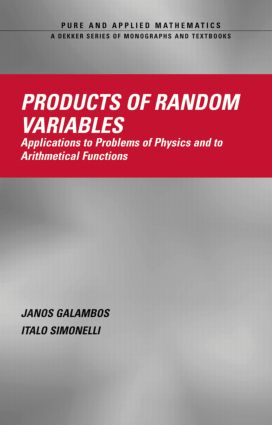Products of Random Variables

Applications to Problems of Physics and to Arithmetical Functions, 1st Edition

CRC Press

344 pages

Purchasing Options:\$ = USD
Hardback: 9780824754020
pub: 2004-07-20
SAVE ~\$60.00
Currently out of stock
\$300.00
\$240.00
x

FREE Standard Shipping!

Description

Products of Random Variables explores the theory of products of random variables through from distributions and limit theorems, to characterizations, to applications in physics, order statistics, and number theory. It uses entirely probabilistic arguments in actualizing the potential of the asymptotic theory of products of independent random variables and obtaining results with dependent variables using a new Bonferroni-type argument.

Systematically and comprehensively tracks the progression of research completed in the area over the last twenty years.

Well-indexed and well-referenced, Products of Random Variables

• Clarifies foundational concepts such as symmetric and limiting distributions of products

• Examines various limit theorems, from logarithmically Poisson distributions to triangular arrays

• Explores characterization theorems, detailing normal, Cauchy, and bivariate distributions

• Describes models of interactive particles

• Elucidates dual systems of interactive particles, dual systems of increasing size, and random walks

• Covers the Kubilius-Turán inequality and distributions for multiplicative functions

• Probes sequences of prime divisors and prime numbers

• Discusses Markov chains, Hilbert spaces, and quotients of random variables

• Presents income growth models and numerous other applied models tapping products of random variables

Authored by eminent scholars in the field, this volume is an important research reference for applied mathematicians, statisticians, physicists, and graduate students in these disciplines.

• Reviews

"This book is the first monograph that is completely devoted to the theory of products of random variables (PRV). It gives the most comprehensive review of the recent results in this area and could be used as a handbook in PRV…the subject index covers the content of the book quite satisfactorily. This book is a useful source of information for specialists in probability theory and/or number theory. This volume will be very useful in any mathematical library."

-Mathematical Reviews, Issue 2005g

Foundations

Limit Theorems

Characterization

Interacting Particles

Arithmetical Functions

Miscellaneous Results

Bibliography

Author Index

Subject Index